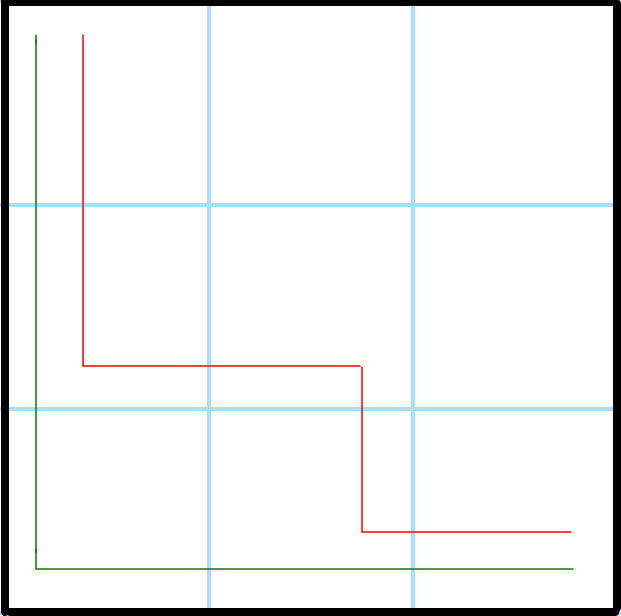# Number of ways you can always stay either above or below the main diagonal

## Introduction:

We are given an integer ‘N’. We need to consider a N * N grid. We are currently at the top left corner of the grid. We need to find the number of ways by which we can reach the bottom right corner if we can make the right(i, j+1) or down(i+1, j) move. There is one condition that we cannot cross the diagonal while making any move.

Let us see a few examples:

Input 1: 3

Output 1: 2

Explanation 1:We can see the 2 paths in the red and green lines.

Input 2: 6

Output 2: 42

Input 3: 4

Output 3: 5

## Approach:

The number of downwards movements will always be greater than or equal to the rightward movements because we can not cross the main diagonal. It is a similar pattern to the Catalan’s number.

Based on the above observation we just need to find the Nth Catalan Number.

Also, let us find the answer for a few of the ‘N’ values.

N = 1 -> 1

N = 2 -> 1

N = 3 -> 2

N = 4 -> 5

N = 5 -> 14

N = 6 -> 42

Answer for N = (1 to 6) is following the Catalan Number Series

Nth Catalan Number (KN) = (2NCN ) / (N + 1), where 2NCN is a binomial coefficient.

Total number of ways = KN

Refer to the below implementation of the above approach to find the number of ways you can always stay either above or below the main diagonal

.

Time Complexity: The time complexity for the above approach is O(N) (where ‘N’ is the input we are given for the matrix dimension) because we are only running an O(N) for loop for finding the Binomial Coefficient.

Space Complexity: The space complexity for the above approach is O(1) as we are using constant space for this solution

## FAQs:

1. How do we get to know that to find the number of ways you can always stay either above or below the main diagonal can be solved using Catalan numbers?
• The number of downwards movements will always be greater than or equal to the rightward movements because we can not cross the main diagonal. It is a similar pattern to the Catalan’s number.

Based on the above observation we just need to find the Nth Catalan Number.

1. What is the time complexity for the approach we used to find the number of ways you can always stay either above or below the main diagonal?
• The time complexity for the above approach is O(N) (where ‘N’ is the input we are given for the matrix dimension) because we are only running an O(N) for loop for finding the Binomial Coefficient.

## Key Takeaways:

In this blog, we have covered the following things:

1. We first discussed how we can find how you can always stay above or below the main diagonal using Catalan numbers.
2. Then we saw how we could implement the Catalan Number.

If you want to learn more about Arrays and want to practice some questions which require you to take your basic knowledge on Arrays a notch higher, then you can visit our Guided Path for Arrays

Until then, All the best for your future endeavors, and Keep Coding.